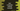# Dart program to find the remainder using remainder method## Use remainder method to find the remainder in dart:

To find the remainder after one number divides another. It is called remainder() and defined in Number class. In this post, I will show you how to use remainder function with an example.

### Definition of remainder:

remainder() function is defined as below:

``Number.remainder(num)``

num is the divisor.

### Return value:

This method returns the remainder of a divisor.

### Example program:

Below is the complete dart program:

``````import 'dart:io';

void main() {
print("Enter the first number : ");

print("Enter the second number : ");

print("Remainder of \$first/\$second \${first.remainder(second)}");
}``````

### Sample Output:

It will give outputs as like below:

``````Enter the first number :
4
Enter the second number :
5
Remainder of 4/5 4

Enter the first number :
10
Enter the second number :
2
Remainder of 10/2 0``````